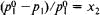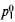# Raoult's Laws

The following article is from The Great Soviet Encyclopedia (1979). It might be outdated or ideologically biased.

## Raoult’s Laws

quantitative relationships that relate the concentration of a solution either to the vapor pressure of the solvent above the solution or to the change in the boiling or freezing point of the solution.

One of F. Raoult’s laws states that the relative lowering of the partial vapor pressure of a solvent is equal to the mole fraction of the solute; that is,whereis the vapor pressure of the pure solvent at a given temperature, p1 is the vapor pressure of the solvent above the solution, and x2 is the mole fraction of the solute. In this form, the law is applicable only to solutions whose saturated vapor behaves as an ideal gas. Solutions for which relation (1) is satisfied at all concentrations and at all temperatures at which the solution can exist are often said to be ideal. In the more general case the fugacities and activities rather than the pressures and concentrations should be used in (1).

For the other Raoult’s law, according to which the elevation of the boiling point (tb) or lowering of the freezing point (tf) of a solution is directly proportional to the molal concentration of the solute, the following relations hold:

(2) ∆tb = Eem ∆tf= Ecm

where ∆tb is the value of the elevation of tb, ∆tf is the value of the lowering of tf m is the molal concentration of the solution, and Ee and Ec are the ebullioscopic and cryoscopie constants of the solvent (these are given in many physicochemical tables). Equations (2) are used to determine the molecular weight of a solute from the experimentally determined values of ∆tb and ∆tf.

M. E. ERLYKINA